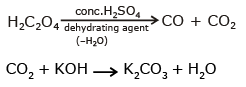Courses

# Test: p-Block Elements- 2

## 30 Questions MCQ Test Chemistry Class 12 | Test: p-Block Elements- 2

Description
This mock test of Test: p-Block Elements- 2 for JEE helps you for every JEE entrance exam. This contains 30 Multiple Choice Questions for JEE Test: p-Block Elements- 2 (mcq) to study with solutions a complete question bank. The solved questions answers in this Test: p-Block Elements- 2 quiz give you a good mix of easy questions and tough questions. JEE students definitely take this Test: p-Block Elements- 2 exercise for a better result in the exam. You can find other Test: p-Block Elements- 2 extra questions, long questions & short questions for JEE on EduRev as well by searching above.
QUESTION: 1

### Which is incorrectly matched ?

Solution: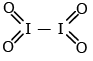It is strictly covalent does not shows cationic & anionic form.

QUESTION: 2

### H3PO2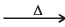(X) + PH3 ; is

Solution: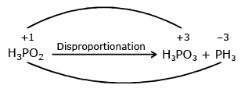QUESTION: 3

### Which of the following species is not a pseudohalide ?

Solution:

Pseudo halides are
CN, NC, OCN, SCN, SeCN
NCS, N3, NCO

QUESTION: 4

An orange solid (X) on heating , gives a colourless gas (Y) and a only green residue (Z). Gas (Y) treatment with Mg, produces a white solid substance...............

Solution:

(NH4)2Cr2O7 → Cr2O3 + H2O + N2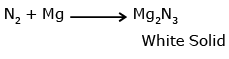QUESTION: 5

Conc. HNO3 is yellow coloured liquid due to

Solution: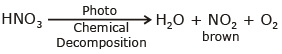Dissolved NO2 makes it yellow

QUESTION: 6

A gas at low temperature does not react with the most of compounds. It is almost inert and is used to create intent atmosphere  in bulbs. The combustion of this gas is exceptionally an endothermic reaction Based on the given information, we can conclude that the gas is.

Solution: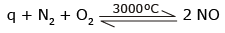N2 is higly stable due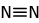and its combustion is endothermic whereas combustion of CO & H2 is exothermic.

QUESTION: 7

when chlorine gas is passed through an aqueous solution of a potassium halide in the presence of chloroform a violet colouration is obtained. On passing more of chlorine water, the violet colour is disappeared and solution becomes colourless. This test confirms the presence of ........ in aqueous solution.

Solution:

Cl2 + KI → K Cl + I2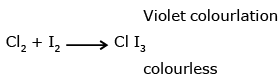QUESTION: 8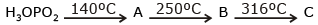Compound (C) is

Solution:QUESTION: 9

An explosive compound (A) reacts with water to produce NH4OH and HOCl. Then, the compound (A), is

Solution: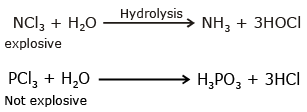QUESTION: 10

An inorganic salt (A) is decomposed at about 523 K to give products (B) and (C). Compounds (C) is a liquid at room temperature and is neutral to limus paper while oxide (B) on burning with white phosphorous given a dehydrating agent (D). Compounds (A), (B), (C) and (D) will be identified as N2O.

Solution: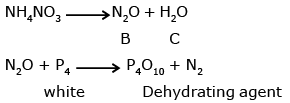QUESTION: 11

An inorganic compound (A) made of two most occurring elements into the earth crust, having  polymeric tetra-hedral net word structure. With carbon. compound (A) produces a poisonous gas (B) which is the most stable diatomic molecule. Compounds (A) and (B) will be

Solution:

SiO2 - sp3
sillica is most abundant in earthiness oxygen atom is sp3 hybridised.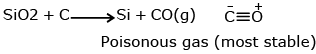QUESTION: 12

A sulphate of a metal (A) on heating evolves two gases (B) and (C) and an oxide (D). Gas (B) turns K2Cr2O7 paper green while gas (C) forms a trimer in which there is non S-S bond. Compounds (D) with HCl, forms a Lewis base (E) which exists as a dimer. Compounds (A), (B)  (C) , (D) and (E) are respectively

Solution: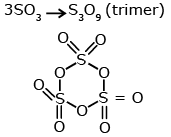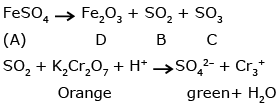QUESTION: 13

A tetra atomic molecule (A) on reaction with nitrogen (I) oxide, produces two substances (B) and (C) ,(B) is a dehyderating agent in its monometric form while substance (C) is a diatomic gas which shows almost intert behavior. The substances (A) and (B) and (C) respectively will be

Solution:

P4 + N2O → P4O10 + N2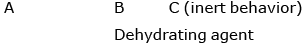QUESTION: 14

First compound of intert gases was prepared by scientist Neil Barthleta in 1962. This compound is

Solution: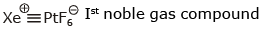QUESTION: 15

Carbon give has X% of CO2 and is used as an antidote for poisoning of Y. Then, X and Y are

Solution:

C + 2O2 → 2CO + CO2
1 mole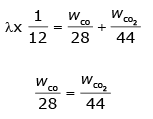QUESTION: 16

The correct order of acidic strength of oxide of nitrogen is

Solution:

Fajan’s rule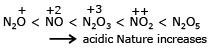QUESTION: 17

Nitrogen dioxide is dissolved in water to produce

Solution:

NO2 + H2O → HNO3 + HNO2
Mixed anhydride

QUESTION: 18

Consider two reactions
I. Zn + conc. HNO3 (hot) → Zn(NO3)2 + X + H2O
II. Zn + dil. HNO3 (cold) → Zn(NO3)2 + Y + H2O

Compounds X and Y are respectively

Solution: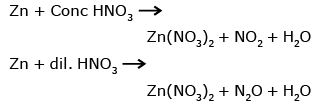QUESTION: 19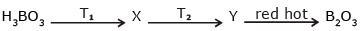it T1 < T2 ten X and Y respectively are

Solution: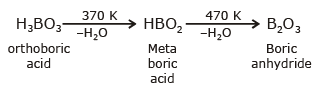QUESTION: 20

Boron forms BX3 type of halides. The correct increasing order of Lewis - acid strength of these halides is

Solution: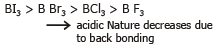QUESTION: 21

Which one of the following compounds on strong heating evolves ammonia gas ?

Solution:

(NH4)2SO4 → NH3 + H2SO4
HNO3 → H2O + NO2 + O2
(NH4)2Cr2O7 → N2 + Cr2O3 + H2O
NH4NO3 → N2O + H2O

QUESTION: 22

The compound (SiH3)3N is

Solution: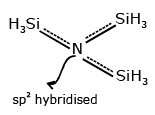QUESTION: 23

The correct order of acid strength of oxyacids of chlorine is

Solution: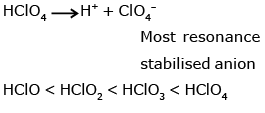QUESTION: 24

In a molecule of phosphorus (V) oxide, there are

Solution: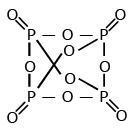QUESTION: 25

The structures of O3 and N3 are

Solution: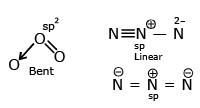QUESTION: 26

When conc. H2SO4 was treated with K4[Fe(CN)6], CO gas was evolved. By mistake, somebody used dilute H2SO4 instead of conc. H2SO4 then the gas evolved was

Solution:

K4[Fe(CN)6] + H2SO4 + H2O → K2SO4 + FeSO4 + (NH4)2SO4 + CO
K4[Fe(CN)6]+dil.H2SO4 → K2SO4 + FeSO4 + HCN

QUESTION: 27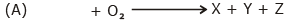(organic Compound)

Compound (A) in pure form does not give ppt. with AgNO3 solution. A mixture containing 70% of (A) and 30% of ether is used as an anaesthetic. Compound (X) and (Y) are oxides while (Z) is a pungen smelling gas. (X) is a neutral oxide which turns cobalt chloride paper pink. Compound (Y) turns liime water milky and produces an acidic solution with water. Compounds (A), (X), (Y) and (Z) respectively will be

Solution:

CHCl3 + AgNO3 → No ppt.
used as anaesthetic
CHCl3 + O2 → H2O + CO2 + Cl2

QUESTION: 28

An inorganic white crystalline compound (A) has a rock salt structure (A) on reaction with conc. H2SO4 and MnO2, evolves a pungent smelling, greenish-yellow gas (B). Compound (A) gives white ppt. of (C) with AgNO3 solution. Compounds (A), (B) and (C) will be respectively

Solution: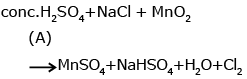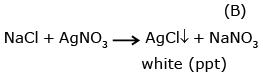QUESTION: 29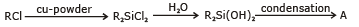Compound (A) is

Solution: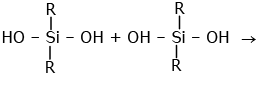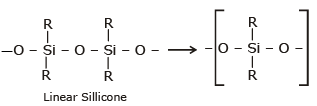QUESTION: 30

When oxalic acid reacts with conc. H2SO4, two gases produced are of neutral and acidic in nature respectively. Potassium hydroxide absorbs one of the two gases. The product formed during this absorption and the gas which gets absorbed are respectively

Solution: